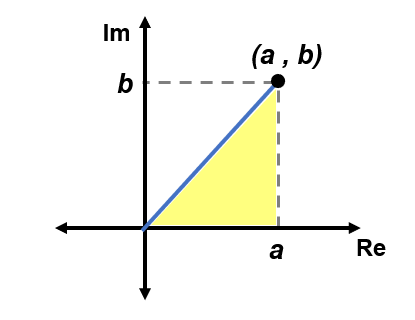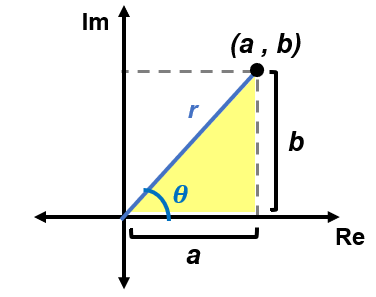# Complex Numbers

## Polar Form Terminology

The form in which we usually see complex numbers in is called rectangular form:

$$z=a+bi$$,

where $$a,b$$ are real numbers and $$i=\sqrt{-1}$$.

However, we can also represent complex numbers in polar form:

$$z=r(cos\theta+isin\theta)$$,

where $$r$$ is called the modulus and $$\theta$$ is called the argument of the complex number.

Sometimes, you might see $$z=r(cos\theta+isin\theta)$$ abbreviated as $$z=rcis\theta$$.

We will explain how to derive this form and convert between Rectangular and Polar form in the below sections.

## Rectangular Form to Polar Form

Recall that we can represent complex numbers graphically on the complex plane (see Graphing Complex Numbers):

Notice that if we draw a line connect the origin $$(0,0)$$ to the point representing our complex number $$(a,b)$$, then draw another line from that point down to the Real axis, we can form a right-angled triangle:We can then label this magnitude/length of the line connecting the origin to the point $$(a,b)$$ as $$r$$ (modulus) and the angle (argument) formed between that line and the Real axis as $$\theta$$:Finally, we can use trigonometry to help us derive the formulas to calculate the modulus and argument.

Modulus

We can apply the Pythagorean Theorem to the right-angled triangle we identified above:

$$a^2+b^2=r^2$$

We can then rearrange this equation and isolate for $$r$$, which gives us the formula to find the modulus of a complex number given its rectangular form (i.e. $$a+bi$$):

$$r=\sqrt{a^2+b^2}$$

*Note: Sometimes, you may see $$r$$ equal to or expressed as $$|z|$$ since the above formula is the same as the one for finding the magnitude of a complex number.

Tip: Since $$r$$ represents the distance between two points, it must be a positive real number, so when calculating for this value, you can double check your work by making sure $$r \geq 0$$!

Argument

Recall the basic trigonometric ratio for tangent (you can further review this here):

$$tan(\theta)=\frac{opposite}{adjacent}$$

Applying these ratios to the above right-angled triangle gives:

$$tan(\theta)=\frac{b}{a}, \ a\ne 0$$

Then, isolating for $$\theta$$ gives:

$$\theta=tan^{-1}(\frac{b}{a}), \ a\ne 0$$

If it's not specified, then you can choose to express the argument in radians or degrees.

*Note: Since the $$tan(\theta)$$ function has a period of $$\pi$$, you will encounter two possible values for $$\theta$$. To determine which angle is the one you're looking for, identify the quadrant where the plotted point representing the complex number would be.

Example: Convert $$z=-3-3i$$ to polar form.

See the below video for the solution!

## Polar Form to Rectangular Form

To convert from polar form to rectangular form, we can use the right-angled triangle on the complex plane that we mentioned in the above section again.Recall the sine and cosine trigonometric ratios:

$$sin(\theta)=\frac{opposite}{hypotenuse}, \ \cos(\theta)=\frac{adjacent}{hypotenuse}$$

Applying these ratios to the right-angled triangle gives:

$$sin(\theta)=\frac{b}{r}, \ \cos(\theta)=\frac{a}{r}$$

Finally, we can isolate for $$a$$ and $$b$$ by multiplying both sides of each equation by $$r$$:

$$a=rcos(\theta), \ b=rsin(\theta)$$

*Note: In addition to representing complex numbers with (Re, Im)-coordinates, using the two equations we derived above, we can also express them with polar coordinates: $$(rcos(\theta), rsin(\theta))$$.

Example: Convert $$z=3cis(\frac{\pi}{6})$$ into rectangular form.

Solution:

1. We need to determine the modulus and argument of the complex number. In this case, we have:

$$r=3,\ \theta=\frac{\pi}{6}$$

2. Substitute $$r$$ and $$\theta$$ into the two formulas to solve for $$a$$ and $$b$$:

$$a=3cos(\frac{\pi}{6})$$

$$b=3sin(\frac{\pi}{6})$$

3. Evaluate the values of $$a$$ and $$b$$, then substitute them into the rectangular form:

$$a=3(\frac{\sqrt{3}}{2})=\frac{3\sqrt{3}}{2}$$

$$b=3(\frac{1}{2})=\frac{3}{2}$$

$$\therefore z=3cis(\frac{\pi}{6})$$ in rectangular form is $$z=\frac{3\sqrt{3}}{2}+\frac{3}{2}i$$.

## Practice

Time for you to try it out!